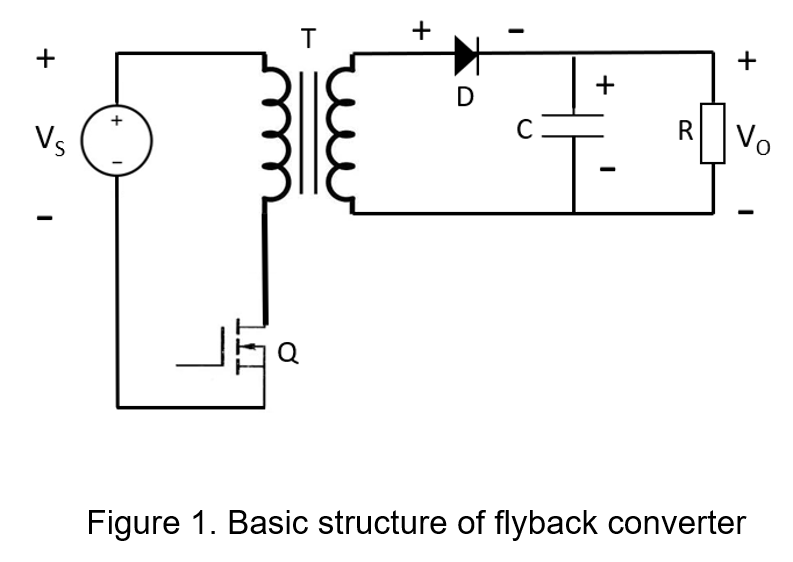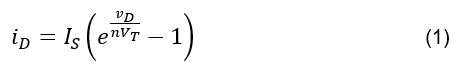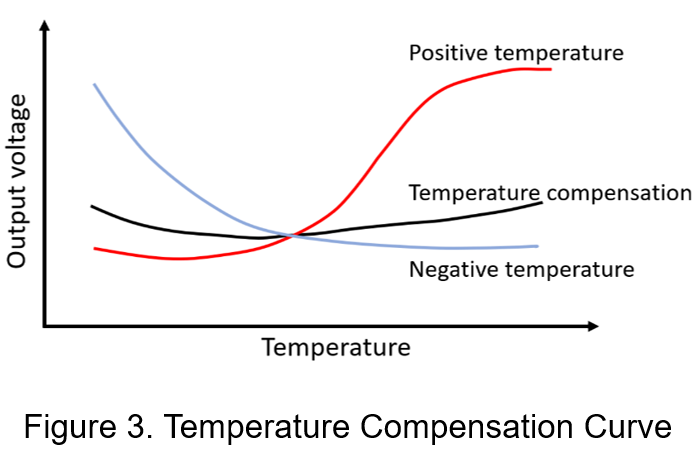# The Influences of Temperature for Converter

Power converters need to have both compact size and high efficiency, but the smaller the size, the poorer the heat dissipation effect, and the higher the temperature, the lower the output conversion efficiency. This article focuses on the influence of temperature on the power converter, analyzes the degree to which component is affected by temperature and actually tests the characteristics of the power converter, and finally explains the concept of temperature compensation to eliminate the influence of temperature on the output characteristics.

1. Relationship Between Power Converter and Temperature

The characteristic of components in power converter varies due to the operating temperature, which can affect the stability. The variation of operating temperature must affect different type of components, and even the temperature characteristic of the same components also will be affected because of different process parameters. As shown in Figure 1, the basic structure of isolated flyback converter uses components such as diodes, capacitors and transformers.The temperature influences of the internal components at different temperatures are as follows:

(1) Diode:

The change of voltage drop and current drop across diode are the function of temperature, as shown in equation (1), where iD and vD are the diode terminal current and terminal voltage, respectively, and IS is the reverse saturation current, VT is the temperature equivalent voltage.Potential barrier voltage decreases when increasing operating temperature, for example silicon diodes decrease by about 2.5mV/°C, germanium diodes decrease by about 1mV/°C

(2) Copper foil on printed circuit boards:

Under the same copper foil thickness, the maximum current carrying capacity of the copper foil is proportional to the temperature.

(3) Capacitor:

Most of the commonly used types of capacitors, such as ceramic capacitors, electrolytic capacitors, mica capacitors, etc., which have high stability against operating temperature variation.

(4) Transformer:

Insensitive to temperature changes, but high temperature can reduce the insulation resistance and service life.

All of the above components are subject to temperature-related disturbances in the output voltage of the power converter. Therefore, it is possible to observe the relationship between the output voltage and the operating temperature by experimental results.

2. Output Voltage and Temperature Relationship Curve

Experimentation on the output voltage versus operating temperature curve of power converters, the following specifications.

 Input voltage 24 Vdc Output voltage 12 Vdc Load current 0.5A Ambient temperature 40℃-90℃ Package 1x1”

The power converter is placed in a programmable temperature and humidity chamber, and when ambient temperature is changed in fixed load current to observe the variation of output voltage.

When the ambient temperature rises, the output voltage of power converter drops due to the different temperature-sensitivity of the internal components in Figure 2. The experimental result shows a negative slope curve in the relationship between temperature and output voltage, and then the slope is calculated to be approximately -0.3mV/°C.3. Temperature Compensation Concept

High temperature causes to increase the bias current of power converter slightly, which causes the negative temperature slope becomes significantly larger in experimental results. In order that the output voltage does not change with different temperature, a temperature compensation circuit is necessary. The temperature compensation circuit adds a positive temperature coefficient element, and the voltage across the element is proportional to the temperature, so the curve of temperature and output voltage has a positive slope relationship. If combining the original negative temperature coefficient components, a temperature-independent correlated output voltage can be realized, as shown in Figure 3.The temperature compensation circuit of the power converter uses specific resistors to bias, which generates a voltage that is proportional to the absolute temperature, so it can cancel the influence of negative temperature coefficient of the diode's forward conduction voltage by negative feedback amplifier. Therefore, how to select suitable resistors to generate positive temperature-coefficient current is a key in the temperature-compensation circuit.

In another case, If the base current of BJT in circuit becomes larger as the temperature rises, temperature compensation circuit can be used the both of resistive divider and diode to generate a negative temperature coefficient current to compensate for the positive temperature characteristics of the base current. As the result, the base voltage is not affected by operating temperature to stabilize base current.

4. Conclusion

In the pursuit of high efficiency and small chip-area, it is important to consider the effect of operating temperature on the power converter. This article discusses the temperature influences of the common components in different temperatures, and confirms from the measurement results that when the temperature rises continuously, the output voltage of the power converter drops slowly, resulting in the conversion efficiency decreases. Therefore, using the temperature compensation circuit is helpful directly to the stability of the output voltage.

CTC is a professional service provider for high-end power supply modules (AC to DC Converter and DC to DC Converter) for critical applications worldwide since 30 years. Our core competence is to design and deliver products with leading technologies, competitive pricing, extremely flexible lead-time, global technical service and high-quality manufacturing (Made In Taiwan).

CTC is the only corporation certificated with ISO-9001, IATF-16949, ISO22613(IRIS), and ESD/ANSI-2020. We can 100% ensure not only the product, but also our workflow and service to match quality management system for every high-end application from the very beginning. From design to manufacturing and technical support, every single detail is operated under highest standard.